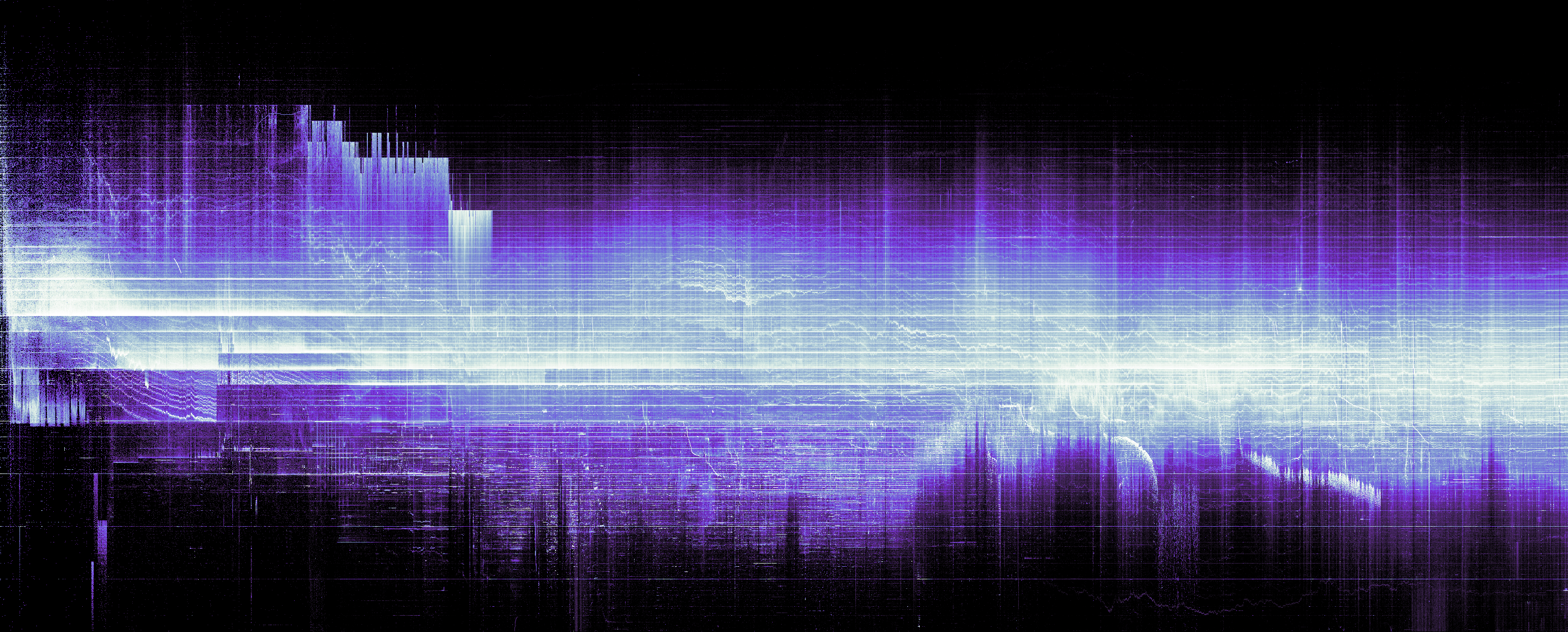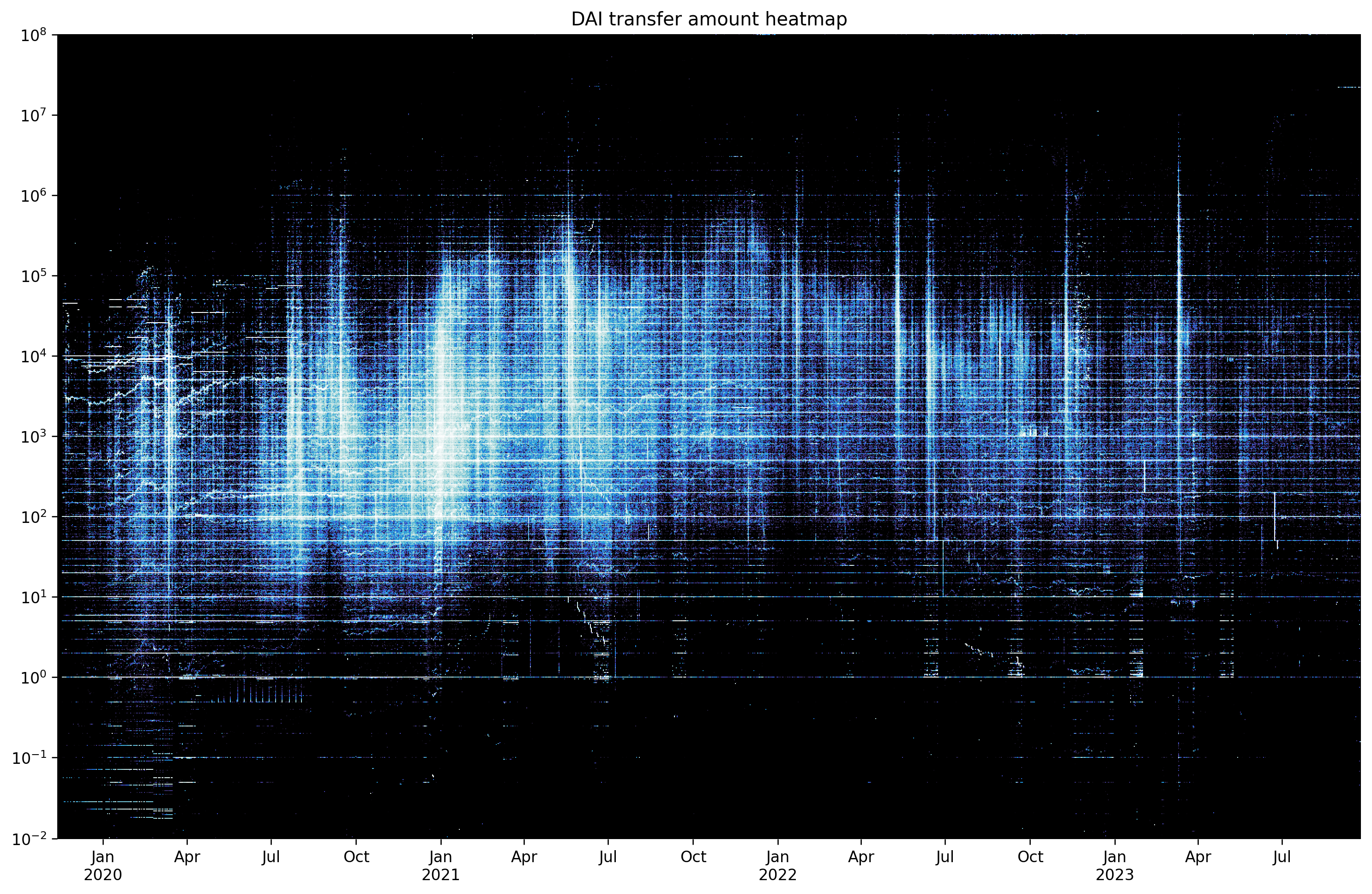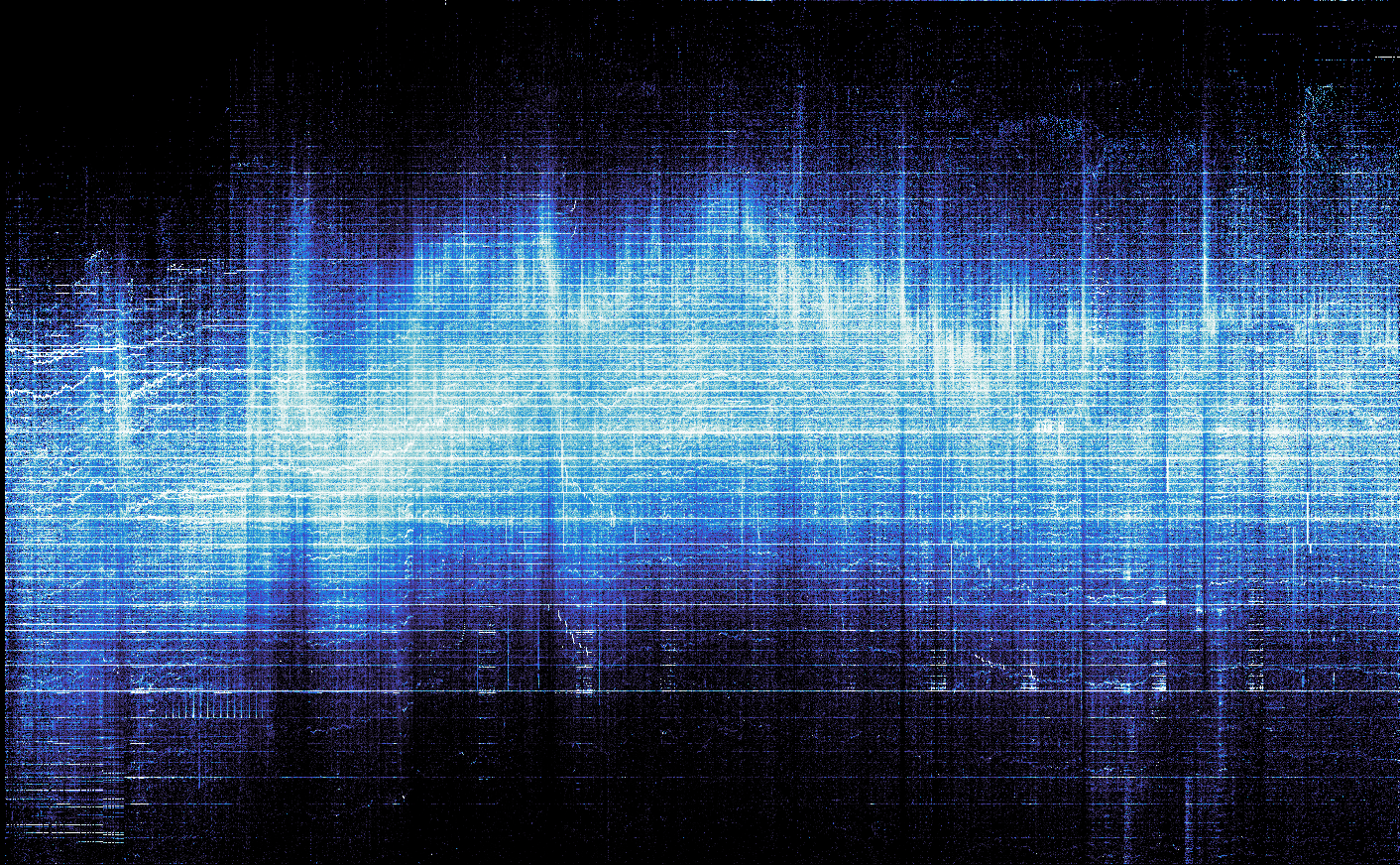# Ethereum Transfers Heatmap

Observe hidden patterns in token transfers

ethereum
data
Author

banteg

Published

September 27, 2023

This work is heavily inspired by the amazing visualizations of bitcoin inputs/outputs at utxo.live.

I’ve replicated it by plotting 2.1 billion native ether transfers.This post will apply the same method to ERC20 token transfers, so you can follow along with your favortite token and without needing a large transfers dataset.

We will be using `jupyter`, `cryo`, `polars`, `matplotlib`, `datashader`, `altair`. You will learn how to render billions of data points and how to display them properly.

## Ethereum data

We’ll make a heatmap of amount distribution among 18.3 million DAI transfers on Ethereum mainnet.

If you want a larger dataset, you can try USDC (73.3 million), BTC UTXO (129 million), USDT (206 million transfers) or native ether transfers (2.1 billion).

``````contract_address = "0x6b175474e89094c44da98b954eedeac495271d0f"
amount_scale = 1e18``````

If you have `cryo logs` (125 GB) dataset available, you can slice a subset from it, filtering by `contract_address` and `topic0`.

You can also get it ad hoc using cryo. We only require `block_number` and `data` columns, with `data` encoding the amount sent.

If you want to plot native ether transfers, you would need `cryo transactions` (304 GB) dataset.

Imports and configs
``````import cryo
import numpy as np
import polars as pl
import cmasher
from IPython import display

pl.Config.set_tbl_hide_column_data_types(True)
pl.Config.set_tbl_hide_dataframe_shape(True)``````
``````from eth_utils import decode_hex

raw_transfers = (
pl.scan_parquet("~/data/logs/*.parquet")
.filter(
& (pl.col.topic0 == decode_hex(topic0))
)
.select("block_number", "data")
)``````
``````cryo.freeze(
"logs",
topic0=topic0,
align=True,
chunk_size=100000,
columns=["block_number", "data"],
sort=["block_number"],
output_dir="cryo_logs",
)
raw_transfers = pl.scan_parquet("cryo_logs/*.parquet")``````
``````raw_transfers = (
pl.scan_parquet("~/data/transactions/*.parquet")
.filter(pl.col.value != "0")
.with_columns(amount=pl.col.value.str.to_decimal().cast(pl.Float64()) / 1e18)
.select("block_number", "amount")
)``````

First, export the outstanding coins with bitcoin-utxo-dump.

``bitcoin-utxo-dump -f height,amount``

Then, extract block timestamps from LevelDB using python-bitcoin-blockchain-parser and join them with the coins.

``````from os.path import expanduser
from blockchain_parser.blockchain import Blockchain

blocks = []
blockchain = Blockchain(expanduser("~/.bitcoin/blocks"))

for block in blockchain.get_ordered_blocks(expanduser("~/.bitcoin/blocks/index")):

blocks = pl.DataFrame(blocks, schema=["height", "timestamp"])

decoded_transfers = (
utxo.join(blocks, on="height").sort("height").rename({"height": "block_number"})
)``````

Decode the amounts from `data` column using `map_batches`. If your machine can’t fit the whole column into memory, you can try your luck with `map_elements`.

``````def decode_amounts(series):
return pl.Series(
"amount",
[int.from_bytes(item, "big") for item in series],
dtype=pl.Float64,
)

decoded_transfers = raw_transfers.select(
"block_number",
amount=pl.col.data.map_batches(decode_amounts) / amount_scale,
).collect()``````

## Heatmap size

We need to decide on the heatmap size early, because the bucket sizes would depend on it. Let data inform our decision.

The x axis would show the timestamp, so we need to know the date range to pick an appropriate interval.

To do this, we look up timestamps from `cryo blocks` (0.86 GB) dataset.

``````blocks = (
pl.scan_parquet("~/data/blocks/*.parquet")
.select(
block_number="number",
timestamp=pl.from_epoch("timestamp"),
)
.collect()
)
decoded_transfers = decoded_transfers.join(blocks, on="block_number")
print(len(decoded_transfers), 'transfers')``````
``18348925 transfers``
``````interval = "1d"

time_span = decoded_transfers.select(
min=pl.col.timestamp.first(),
max=pl.col.timestamp.last(),
).with_columns(
pl.all().dt.truncate(interval).suffix("_t"),
span=pl.col.max - pl.col.min,
)
time_range = pl.datetime_range(
time_span[0, "min_t"], time_span[0, "max_t"], interval, eager=True
)
time_span.select("min", "max", "span")``````
min max span
2019-11-13 21:22:33 2023-09-24 09:34:59 1410d 12h 12m 26s

The data spans 1410.5 days, a 1 day interval sounds fitting. We generate a datetime range to avoid any off-by-one errors later.

For the y axis, we would likely want a log scale. We look up the range in the data, but choose the extents ourselves.

``````amount_span = decoded_transfers.filter(pl.col.amount > 0).select(
min=pl.col.amount.min(),
max=pl.col.amount.max(),
min_log=pl.col.amount.min().log(10),
max_log=pl.col.amount.max().log(10),
)
amount_span``````
min max min_log max_log
1.0000e-18 5.4545e8 -18.0 8.736753
``````golden = (1 + 5**0.5) / 2
aspect = golden
width = len(time_range)
height = int(width / aspect)
buckets = np.logspace(-2, 8, height)
width, height``````
``(1412, 872)``

## Heatmap data

Polars has a very handy `cut` method for binning values into buckets. We count the number of transfers with the amount falling in every bucket on each day.

For amounts exceeding the bucket range, polars uses a `float("inf")` break, which wouldn’t work with `datashader`. We fix this in the last step, as well as zero out the lowest bucket, as it includes all lower values, which makes it not very useful.

``````transfer_stats = (
decoded_transfers.with_columns(
bucket=pl.col.amount.cut(buckets, include_breaks=True),
)
.sort("timestamp")
.group_by_dynamic("timestamp", every=interval)
.agg(
txs_per_bucket=pl.col.bucket.struct.field("brk").value_counts(),
)
.explode("txs_per_bucket")
.unnest("txs_per_bucket")
.rename({"brk": "bucket", "counts": "num_txs"})
.with_columns(
bucket=pl.when(pl.col.bucket == float("inf"))
.then(buckets[-1])
.otherwise(pl.col.bucket),
num_txs=pl.when(pl.col.bucket == buckets).then(0).otherwise(pl.col.num_txs),
)
)
print(len(transfer_stats), "datapoints")``````
``837412 datapoints``

## Matplotlib

With these data points we can plot the data. You can’t really use your regular charting methods when dealing with millions of points.

But what is a heatmap if not a colormapped raster image? We could try to construct the image from our dense data, looking up x coordinates from `time_range` and y coordinates from `buckets`.

To add the properly labeled ticks, we do a reverse translation from x and y coordinates to timestamp and buckets. We would override the tick labels with our computed values.

To render the image, we could use something like `imshow` from `matplotlib`. It will appear very dim, so you would want to adjust the data or do some colormap normalization1.

• More search might bring a different approach with histogram equalization2 from `scikit-image` to finally arrive at a decent result.

• If you try to add the axes, you would quickly learn that matplotlib was not designed to deal with exact dimensions. For some reason, it measures everything in inches. Moreover, if you try to rescale the axes, it would squish the image, and we want to blip the heatmap on the chart as is. There is `plt.figimage`, but it doesn’t work with 2x resolution and you would need to calculate the offset (in pixels this time) by hand.

Below I present an easier method, which relies on an obscure combination of options. If you create a figure the size of the image and then add the axes with zero margin, the image would be pixel perfect, but the axes would be outside frame. When saving an image, you can set `bbox_inches='tight'` and matplotlib would expand the output image to reveal the axes.

See matplotlib code
``````from matplotlib import pyplot as plt
from skimage.exposure import equalize_hist

%config InlineBackend.figure_format = 'retina'

# convert timestamps to x coordinates and buckets to y coordinates
date_to_x = (
pl.DataFrame({"timestamp": time_range})
.with_row_count()
.rename({"row_nr": "x"})
)
bucket_to_y = (
pl.DataFrame({"bucket": buckets})
.with_row_count()
.rename({"row_nr": "y"})
)
source = (
transfer_stats
.join(date_to_x, on="timestamp")
.join(bucket_to_y, on="bucket")
)
# convert datapoints to image
x = source["x"].to_numpy()
y = source["y"].to_numpy()
w = source["num_txs"].to_numpy()
H, xe, ye = np.histogram2d(x, y, weights=w, bins=[width, height])
# match the figure size with the image size
dpi = 120
fig = plt.figure(figsize=(width / dpi, height / dpi), dpi=dpi)
# note: histogram2d flips the cartesian coordinates
im = plt.imshow(equalize_hist(H.T), origin="lower", cmap=cmasher.freeze)
ax = plt.gca()
# y axis ticks
y_ticks = np.logspace(-2, 8, 11)
y_indexes = [np.where(buckets >= t) for t in y_ticks]
ax.set_yticks(y_indexes, [f'\$10^{{{int(np.log10(n))}}}\$' for n in y_ticks])
# x axis ticks
dts = date_to_x.group_by_dynamic('timestamp', every='1q').agg(pl.col.x.first()).tail(-1)
x_ticks = dts['timestamp'].cast(pl.Date).to_list()
ax.set_xticks(dts['x'].to_numpy(), [f'{x.strftime("%b")}' + (f'\n{x.strftime("%Y")}' if x.month == 1 else "") for x in x_ticks])
plt.title('DAI transfer amount heatmap')
# this method works both for `show` and `savefig`
plt.savefig('heatmap-matplotlib.png', bbox_inches='tight')
plt.show()``````But what if I told you there is a better way, which does much of this by default.

You know the devs are onto something when their tutorial starts from a chapter-long rant3 about all the bad charts people are making.

• Their peers also have strong words (and a paper4) about colormaps matplotlib ships with. Instead, they recommend using a perceptually uniform colormap. Two such collections are colorcet and cmasher, the latter offers more artistic options.

• We use `datashader` here, since it offers a pipeline flexible enough to render a billion points on a laptop.

First, convert the timestamps to float as required by `xarray`. Normalize the transaction counts by day using a window function so the image is more legible. If you want to use raw counts, change `ds.sum("normalized")` to `ds.sum("num_txs")`.

Next, create a canvas and add the data points there. Datashader supports aggregation, so you can simply halve `plot_width` and `plot_height` and it would render a preview image for you. Fill untouched buckets with zeros, otherwise they would appear as transparent.

Verify the dimensions are correct using `ds.count` aggregation. If you see horizontal or vertical streaks, your sizes do not match up.

Finally, apply a colormap using `tf.shade`. It would normalize the values to better translate them to the colormap. By default, datashader uses histogram equalization `how="eq_hist"`, a nice choice for a large dataset. It would spread out the values in a way that would occupy the full range of the colormap, bringing out the most contrast. Other options include `linear` (matplotlib default), logarithmic `log`, and cube root `cbrt`.

``````import datashader as ds
from datashader import transfer_functions as tf

df = transfer_stats.with_columns(
pl.col.timestamp.cast(pl.Float64),
normalized=pl.col.num_txs / pl.col.num_txs.sum().over("timestamp"),
).to_pandas()

canvas = ds.Canvas(plot_width=width, plot_height=height, y_axis_type="log")
points = canvas.points(df, "timestamp", "bucket", agg=ds.sum("normalized")).fillna(0)
heatmap.to_pil().save("heatmap.png")
display.Image("heatmap.png")``````## Altair

We have plotted 837,412 data points faster than you blinked. And datashader did a much better job than matplotlib utilizing the full range of the colormap.

Below I will show another way of adding axes to the image using altair.

Altair excels at layering and combining charts. We plot two points: one with the minimum coordinates where we blip the image, and another invisible point at the maximum coordinates. The chart size is halved so it renders better on a retina screen.

To render an image in altair, you specify an url, which doesn’t work with local images in a local jupyter easily. The workaround is to embed a base64-encoded image in the source data.

The upside of this approach is you have real axes now, which you can allow altair to render nicely instead of mapping the values by hand. The chart also looks crisp at any resolution because everything but the heatmap is vector, unlike matplotlib5.

• 5 matplotlib can render svg, but nobody uses this for some reason

• See altair code
``````import io
import base64
import altair as alt

def xarray_to_base64(image):
buffer = io.BytesIO()
image.to_pil().convert("RGB").save(buffer, format="png")
encoded = base64.b64encode(buffer.getvalue())
return "data:image/png;base64," + encoded.decode()

extents = pl.DataFrame(
{
"timestamp": [transfer_stats[0, "timestamp"], transfer_stats[-1, "timestamp"]],
"amount": [buckets, buckets[-1]],
"image_data": [xarray_to_base64(heatmap), None],
}
)

axes = (
alt.Chart(
extents.to_pandas().tail(1),
title="DAI transfer amount heatmap",
width=width // 2,
height=height // 2,
)
.mark_point(opacity=0)
.encode(
alt.X("timestamp:T").axis(tickCount=10, labelExpr="[timeFormat(datum.value, '%b'), timeFormat(datum.value, '%m') == '01' ? timeFormat(datum.value, '%Y') : '']"),
alt.Y("amount:Q").scale(type="log"),
)
)

fill = (
alt.Chart(